# Quick introduction to calculus with Julia

Julia can be downloaded and used like other programming languages.

launch binder

Julia can be used through the internet for free using the mybinder.org service. To do so, click on the CalcululsWithJulia.ipynb file after launching Binder by clicking on the badge.

Here are some Julia usages to create calculus objects.

The Julia packages loaded below are all loaded when the CalculusWithJulia package is loaded.

A Julia package is loaded with the using command:

using LinearAlgebra


The LinearAlgebra package comes with a Julia installation. Other packages can be added. Something like:

using Pkg
Pkg.add("SomePackageName")


These notes have an accompanying package, CalculusWithJulia, that when installed, as above, also installs most of the necessary packages to perform the examples.

Packages need only be installed once, but they must be loaded into each session for which they will be used.

using CalculusWithJulia
using Plots


Packages can also be loaded through import PackageName. Importing does not add the exported objects of a function into the namespace, so is used when there are possible name collisions.

## Types

Objects in Julia are "typed." Common numeric types are Float64, Int64 for floating point numbers and integers. Less used here are types like Rational{Int64}, specifying rational numbers with a numerator and denominator as Int64; or Complex{Float64}, specifying a comlex number with floating point components. Julia also has BigFloat and BigInt for arbitrary precision types. Typically, operations use "promotion" to ensure the combination of types is appropriate. Other useful types are Function, an abstract type describing functions; Bool for true and false values; Sym for symbolic values (through SymPy); and Vector{Float64} for vectors with floating point components.

For the most part the type will not be so important, but it is useful to know that for some function calls the type of the argument will decide what method ultimately gets called. (This allows symbolic types to interact with Julia functions in an idiomatic manner.)

## Functions

### Definition

Functions can be defined four basic ways:

• one statement functions follow traditional mathematics notation:

f(x) = exp(x) * 2x

f (generic function with 1 method)

• multi-statement functions are defined with the function keyword. The end statement ends the definition. The last evaluated command is returned. There is no need for explicit return statement, though it can be useful for control flow.

function g(x)
a = sin(x)^2
a + a^2 + a^3
end

g (generic function with 1 method)

• Anonymous functions, useful for example, as arguments to other functions or as return values, are defined using an arrow, ->, as follows:

fn = x -> sin(2x)
fn(pi/2)

1.2246467991473532e-16


In the following, the defined function, Derivative, returns an anonymously defined function that uses a Julia package, loaded with CalculusWithJulia, to take a derivative:

Derivatve(f::Function) = x -> ForwardDiff.derivative(f, x)    # ForwardDiff is loaded in CalculusWithJulia

Derivatve (generic function with 1 method)


(The D function of CalculusWithJulia implements something similar.)

• Anonymous function may also be created using the function keyword.

For mathematical functions $f: R^n \rightarrow R^m$ when $n$ or $m$ is bigger than 1 we have:

• When $n =1$ and $m > 1$ we use a "vector" for the return value

r(t) = [sin(t), cos(t), t]

r (generic function with 1 method)


(An alternative would be to create a vector of functions.)

• When $n > 1$ and $m=1$ we use multiple arguments or pass the arguments in a container. This pattern is common, as it allows both calling styles.

f(x,y,z) = x*y + y*z + z*x
f(v) = f(v...)

f (generic function with 2 methods)


Some functions need to pass in a container of values, for this the last definition is useful to expand the values. Splatting takes a container and treats the values like individual arguments.

Alternatively, indexing can be used directly, as in:

f(x) = x*x + x*x + x*x

f (generic function with 2 methods)

• For vector fields ($n,m > 1$) a combination is used:

F(x,y,z) = [-y, x, z]
F(v) = F(v...)

F (generic function with 2 methods)


### Calling a function

Functions are called using parentheses to group the arguments.

f(t) = sin(t)*sqrt(t)
sin(1), sqrt(1), f(1)

(0.8414709848078965, 1.0, 0.8414709848078965)


When a function has multiple arguments, yet the value passed in is a container holding the arguments, splatting is used to expand the arguments, as is done in the definition F(v) = F(v...), above.

### Multiple dispatch

Julia can have many methods for a single generic function. (E.g., it can have many different implementations of addiion when the + sign is encountered.) The types of the arguments and the number of arguments are used for dispatch.

Here the number of arguments is used:

Area(w, h) = w * h    # area of rectangle
Area(w) = Area(w, w)  # area of square using area of rectangle defintion

Area (generic function with 2 methods)


Calling Area(5) will call Area(5,5) which will return 5*5.

Similarly, the definition for a vector field:

F(x,y,z) = [-y, x, z]
F(v) = F(v...)

F (generic function with 2 methods)


takes advantage of multiple dispatch to allow either a vector argument or individual arguments.

Type parameters can be used to restrict the type of arguments that are permitted. The Derivative(f::Function) definition illustrates how the Derivative function, defined above, is restricted to Function objects.

### Keyword arguments

Optional arguments may be specified with keywords, when the function is defined to use them. Keywords are separated from positional arguments using a semicolon, ;:

circle(x; r=1) = sqrt(r^2 - x^2)
circle(0.5), circle(0.5, r=10)

(0.8660254037844386, 9.987492177719089)


The main (but not sole) use of keyword arguments will be with plotting, where various plot attribute are passed as key=value pairs.

## Symbolic objects

The add-on SymPy package allows for symbolic expressions to be used. Symbolic values are defined with @vars, as below.

using SymPy
@vars x y z  # no comma as done here, though @vars(x,y,z) is also available
x^2 + y^3 + z

\begin{equation*}x^{2} + y^{3} + z\end{equation*}

Assumptions on the variables can be useful, particularly with simplification, as in

@vars x y z real=true

(x, y, z)


Symbolic expressions flow through Julia functions symbolically

sin(x)^2 + cos(x)^2

\begin{equation*}\sin^{2}{\left(x \right)} + \cos^{2}{\left(x \right)}\end{equation*}

Numbers are symbolic once SymPy interacts with them:

x - x + 1  # 1 is now symbolic

\begin{equation*}1\end{equation*}

The number PI is a symbolic pi. a

sin(PI), sin(pi)

(0, 1.2246467991473532e-16)


Use Sym to create symbolic numbers, N to find a Julia number from a symbolic number:

1 / Sym(2)

\begin{equation*}\frac{1}{2}\end{equation*}
N(PI)

π = 3.1415926535897...


Many generic Julia functions will work with symbolic objects through multiple dispatch (e.g., sin, cos, ...). Sympy functions that are not in Julia can be accessed through the sympy object using dot-call notation:

sympy.harmonic(10)

\begin{equation*}\frac{7381}{2520}\end{equation*}

Some Sympy methods belong to the object and a called via the pattern object.method(...). This too is the case using SymPy with Julia. For example:

A = [x 1; x 2]
A.det()   # determinant of symbolic matrix A

## Containers

We use a few different containers:

• Tuples. These are objects grouped together using parentheses. They need not be of the same type

x1 = (1, "two", 3.0)

(1, "two", 3.0)


Tuples are useful for programming. For example, they are uesd to return multiple values from a function.

• Vectors. These are objects of the same type (typically) grouped together using square brackets, values separated by commas:

x2 = [1, 2, 3.0]  # 3.0 makes theses all floating point

3-element Array{Float64,1}:
1.0
2.0
3.0


Unlike tuples, the expected arithmatic from Linear Algebra is implemented for vectors.

• Matrices. Like vectors, combine values of the same type, only they are 2-dimensional. Use spaces to separate values along a row; semicolons to separate rows:

x3 = [1 2 3; 4 5 6; 7 8 9]

3×3 Array{Int64,2}:
1  2  3
4  5  6
7  8  9

• Row vectors. A vector is 1 dimensional, though it may be identified as a column of two dimensional matrix. A row vector is a two-dimensional matrix with a single row:

x4 = [1 2 3.0]

1×3 Array{Float64,2}:
1.0  2.0  3.0


These have indexing using square brackets:

x1, x2, x3

(1, 2.0, 7)


Matrices are usually indexed by row and column:

x3[1,2] # row one column two

2


For vectors and matrices - but not tuples, as they are immutable - indexing can be used to change a value in the container:

x2, x3[1,1] = 2, 2

(2, 2)


Vectors and matrices are arrays. As hinted above, arrays have mathematical operations, such as addition and subtraction, defined for them. Tuples do not.

Destructuring is an alternative to indexing to get at the entries in certain containers:

a,b,c = x2

3-element Array{Float64,1}:
2.0
2.0
3.0


### Structured collections

An arithmetic progression, $a, a+h, a+2h, ..., b$ can be produced efficiently using the range operator a:h:b:

5:10:55  # an object that describes 5, 15, 25, 35, 45, 55

5:10:55


If h=1 it can be omitted:

1:10     # an object that describes 1,2,3,4,5,6,7,8,9,10

1:10


The range function can efficiently describe $n$ evenly spaced points between a and b:

range(0, pi, length=5)  # range(a, stop=b, length=n) for version 1.0

0.0:0.7853981633974483:3.141592653589793


This is useful for creating regularly spaced values needed for certain plots.

## Iteration

The for keyword is useful for iteration, Here is a traditional for loop, as i loops over each entry of the vector [1,2,3]:

for i in [1,2,3]
print(i)
end

123


List comprehensions are similar, but are useful as they perform the iteration and collect the values:

[i^2 for i in [1,2,3]]

3-element Array{Int64,1}:
1
4
9


Comprehesions can also be used to make matrices

[1/(i+j) for i in 1:3, j in 1:4]

3×4 Array{Float64,2}:
0.5       0.333333  0.25      0.2
0.333333  0.25      0.2       0.166667
0.25      0.2       0.166667  0.142857


(The three rows are for i=1, then i=2, and finally for i=3.)

Comprehensions apply an expression to each entry in a container through iteration. Applying a function to each entry of a container can be facilitated by:

• Broadcasting. Using . before an operation instructs Julia to match up sizes (possibly extending to do so) and then apply the operation element by element:

xs = [1,2,3]
sin.(xs)   # sin(1), sin(2), sin(3)

3-element Array{Float64,1}:
0.8414709848078965
0.9092974268256817
0.1411200080598672


This example pairs off the value in bases and xs:

bases = [5,5,10]
log.(bases, xs)  # log(5, 1), log(5,2), log(10, 3)

This example broadcasts the scalar value for the base with xs:

log.(5, xs)

3-element Array{Float64,1}:
0.0
0.43067655807339306
0.6826061944859854


Row and column vectors can fill in:

ys = [4 5] # a row vector
f(x,y) = (x,y)
f.(xs, ys)    # broadcasting a column and row vector makes a matrix, then applies f.

3×2 Array{Tuple{Int64,Int64},2}:
(1, 4)  (1, 5)
(2, 4)  (2, 5)
(3, 4)  (3, 5)


This should be contrasted to the case when both xs and ys are (column) vectors, as then they pair off:

f.(xs, [4,5])
• The map function is similar, it applies a function to each element:

map(sin, [1,2,3])

3-element Array{Float64,1}:
0.8414709848078965
0.9092974268256817
0.1411200080598672


## Plots

The following commands use the Plots package. The Plots package expects a choice of backend. We will use both plotly and gr (and occasionally pyplot()).

using Plots
pyplot()      # select pyplot. Use gr() for GR; plotly() for Plotly

Plots.PyPlotBackend()


Plotting a univariate function $f:R \rightarrow R$

• using plot(f, a, b)

plot(sin, 0, 2pi)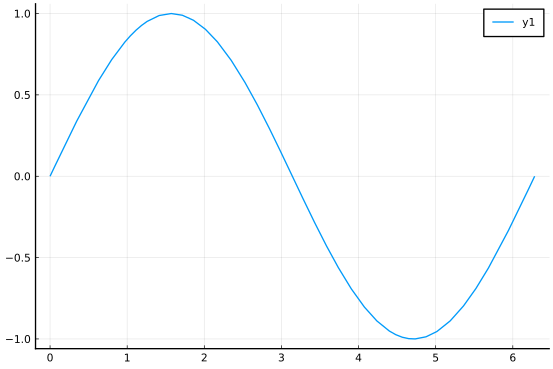Or

f(x) = exp(-x/2pi)*sin(x)
plot(f, 0, 2pi)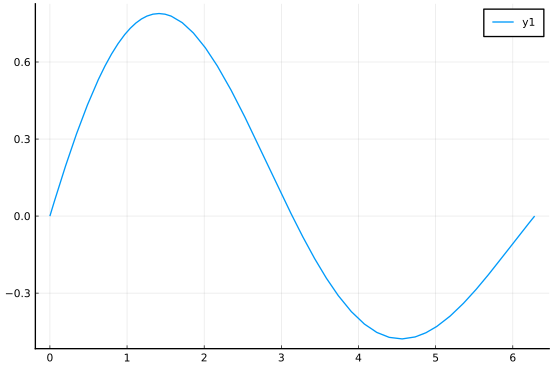Or with an anonymous function

plot(x -> sin(x) + sin(2x), 0, 2pi)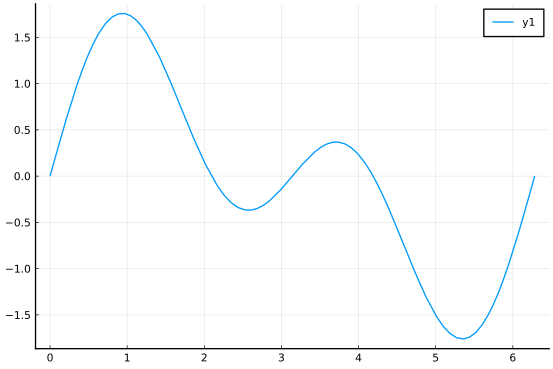Arguments of interest include

AttributeValue
legendA boolean, specify false to inhibit drawing a legend
aspect_ratioUse :equal to have x and y axis have same scale
linewidthIngters greater than 1 will thicken lines drawn
colorA color may be specified by a symbol (leading :).
E.g., :black, :red, :blue
• using plot(xs, ys)

The lower level interface to plot involves directly creating x and y values to plot:

xs = range(0, 2pi, length=100)
ys = sin.(xs)
plot(xs, ys, color=:red)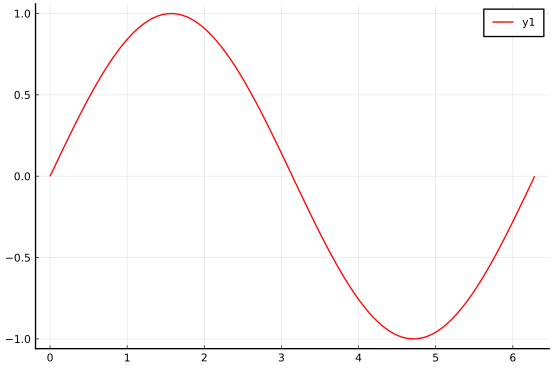• plotting a symbolic expression

A symbolic expression of single variable can be plotted as a function is:

@vars x
plot(exp(-x/2pi)*sin(x), 0, 2pi)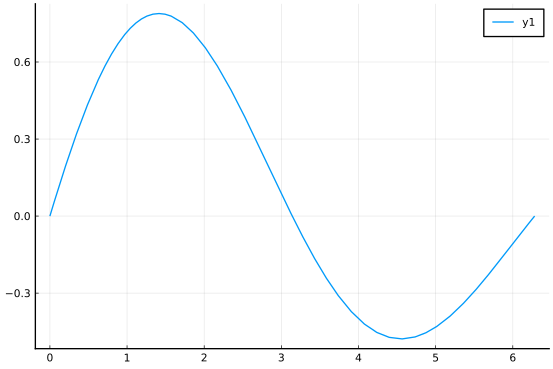• Multiple functions

The ! Julia convention to modify an object is used by the plot command, so plot! will add to the existing plot:

plot(sin, 0, 2pi, color=:red)
plot!(cos, 0, 2pi, color=:blue)
plot!(zero, color=:green)  # no a, b then inherited from graph.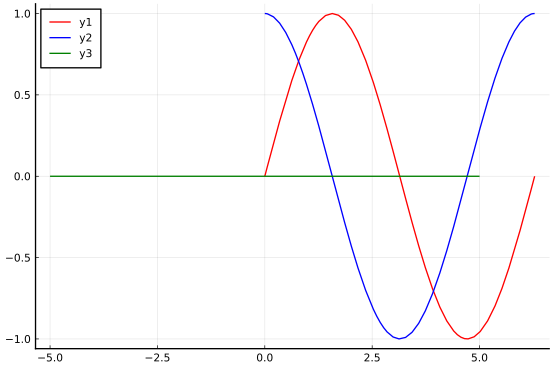The zero function is just 0 (more generally useful when the type of a number is important, but used here to emphasize the $x$ axis).

Plotting a parameterized (space) curve function $f:R \rightarrow R^n$, $n = 2$ or $3$

• Using plot(xs, ys)

Let $f(t) = e^{t/2\pi} \langle \cos(t), \sin(t)\rangle$ be a parameterized function. Then the $t$ values can be generated as follows:

ts = range(0, 2pi, length = 100)
xs = [exp(t/2pi) * cos(t) for t in ts]
ys = [exp(t/2pi) * sin(t) for t in ts]
plot(xs, ys)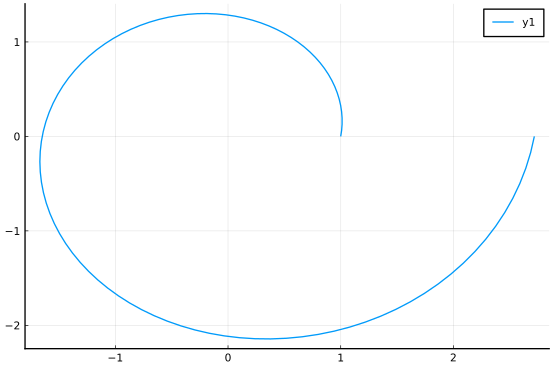• using plot(f1, f2, a, b). If the two functions describing the components are available, then

f1(t) = exp(t/2pi) * cos(t)
f2(t) = exp(t/2pi) * sin(t)
plot(f1, f2, 0, 2pi)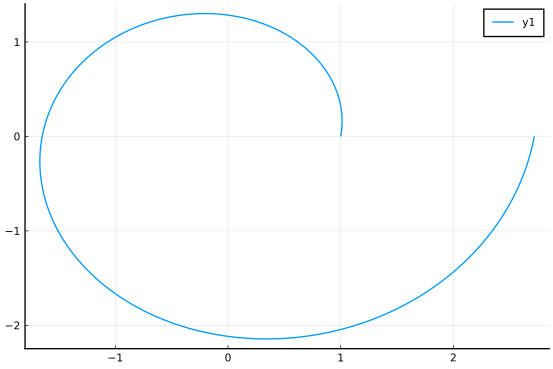• Using plot_parametric_curve. If the curve is described as a function of t with a vector output, then the CalculusWithJulia package provides plot_parametric_curve to produce a plot:

r(t) = exp(t/2pi) * [cos(t), sin(t)]
plot_parametric_curve(r, 0, 2pi)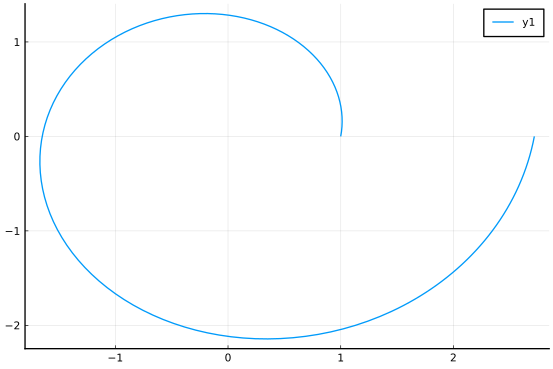The low-level approach doesn't quite work as easily as desired:

ts = range(0, 2pi, length = 4)
vs = r.(ts)

4-element Array{Array{Float64,1},1}:
[1.0, 0.0]
[-0.6978062125430444, 1.2086358139617603]
[-0.9738670205273388, -1.6867871593690715]
[2.718281828459045, -6.657870280805568e-16]


As seen, the values are a vector of vectors. To plot a reshaping needs to be done:

ts = range(0, 2pi, length = 100)
vs = r.(ts)
xs = [vs[i] for i in eachindex(vs)]
ys = [vs[i] for i in eachindex(vs)]
plot(xs, ys)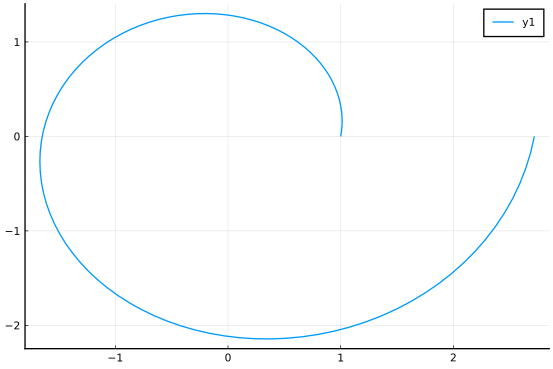This approach is faciliated by the unzip function in CalculusWithJulia (and used internally by plot_parametric_curve):

plot(unzip(vs)...)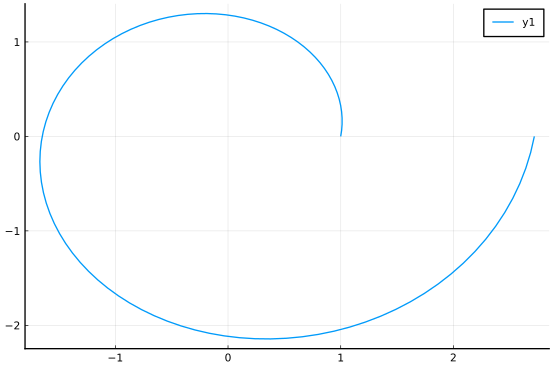• Plotting an arrow

An arrow in 2D can be plotted with the quiver command. We show the arrow(p, v) (or arrow!(p,v) function) from the CalculusWithJulia package, which has an easier syntax (arrow!(p, v), where p is a point indicating the placement of the tail, and v the vector to represent):

gr()
plot_parametric_curve(r, 0, 2pi)
t0 = pi/8
arrow!(r(t0), r'(t0))


The GR package makes nicer arrows that Plotly.

Plotting a scalar function $f:R^2 \rightarrow R$

The surface and contour functions are available to visualize a scalar function of $2$ variables:

• A surface plot

plotly()    # The plotly backend allows for rotation by the mouse; otherwise the camera argument is used
f(x, y) = 2 - x^2 + y^2
xs = ys = range(-2,2, length=25)
surface(xs, ys, f)


The function generates the $z$ values, this can be done by the user and then passed to the surface(xs, ys, zs) format:

surface(xs, ys, f.(xs, ys'))

• A contour plot

The contour function is like the surface function.

contour(xs, ys, f)

contour(xs, ys, f.(xs, ys'))

• An implicit equation. The constraint $f(x,y)=c$ generates an implicit equation. While contour can be used for this type of plot - by adjusting the requested contours - the ImplicitEquations package can as well, and, perhaps. is easier. This package is loaded with CalculusWithJulia; loading it by itself will lead to naming conflicts with SymPy, so best not to do so. ImplicitEquations plots predicates formed by Eq, Le, Lt, Ge, and Gt (or some unicode counterparts). For example to plot when $f(x,y) = \sin(xy) - \cos(xy) \leq 0$ we have:

f(x,y) = sin(x*y) - cos(x*y)
plot(Le(f, 0))     # or plot(f ≦ 0) using \leqq[tab] to create that symbol